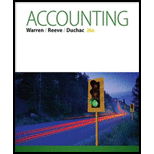Chapter 6, Problem 6.31EX### Accounting (Text Only)

26th Edition
Carl Warren + 2 others
ISBN: 9781285743615

#### Solutions

Chapter
Section### Accounting (Text Only)

26th Edition
Carl Warren + 2 others
ISBN: 9781285743615
Textbook Problem
15 views

# Ratio of sales to assetsKroger, a national supermarket chain, reported the following data (in millions) in its financial statements for a recent year: Total revenue $90,374 Total assets at end of year 23,476 Total assets at beginning of year 23,505 a. Compute the ratio of sales to assets. Round to two decimal places.b. Tiffany & Co. is a large North American retailer of jewelry, with a ratio of sales to assets of 0.92. Why would Tiffany’s ratio of sales to assets be lower than that of Kroger? a. To determine Asset turnover ratio is used to determine the efficiency of the company towards use of asset towards generation of sales. The formula to calculate asset turnover is given below: Assetturnover=NetrevenueAverage total assets The asset turnover for Company K. Explanation Working Notes: Calculate the average total assets. Beginning assets =$23,476

Ending assets = \$23,505

Averagetotalassets=Beginningassets+Ending

b.

To determine

To Comment: Why Company T’s asset turnover is lower than Company K.

### Still sussing out bartleby?

Check out a sample textbook solution.

See a sample solution

#### The Solution to Your Study Problems

Bartleby provides explanations to thousands of textbook problems written by our experts, many with advanced degrees!

Get Started

#### Find more solutions based on key concepts